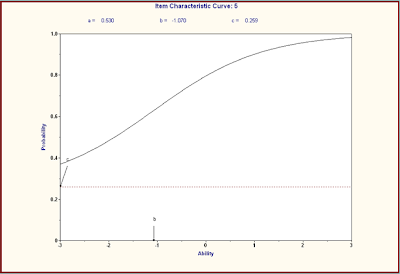## Thursday, January 7, 2010

### So what are item parameters, anyway?

Item Response Theory (IRT) item parameter estimates play an important role in many of the reports generated in Galileo K-12 Online. In addition to describing item characteristics in the Item Parameter report, they help to determine student Development Level (DL) scores and to identify the standards that are recommended for re-teaching efforts in the drill-downs from the Risk Assessment report. But what are they?

The best way to understand what item parameters refer to is to look at an Item Characteristic Curve. On an item characteristic curve, which presents the data for one, specific item, student ability (based on their performance on the assessment as a whole) is plotted on the horizontal axis, with a mean of 0 and a standard deviation of 1. The probability of answering the item correctly is plotted on the vertical axis. Typically the probability of answering correctly is relatively low for low ability students and relatively high for students of higher ability.

The first example (Item #4) is an example of a great item as far as the parameters go. The b-value (difficulty) for that item was 0.689, which is a bit on the difficult side, but not too bad. The important point here is that b-values (item difficulty) are on the same scale as student ability. So what this example is telling us is that students at or above 0.689 standard deviations above the mean are likely to get the answer correct. Students below that point on the ability scale are more likely to answer incorrectly. The b-parameter is also known as the location parameter, because it locates the point on the ability scale where students start demonstrating mastery of the concept.The a-value (discrimination) refers to how well the item discriminates between different ability levels. It’s how steep the rise is in the curve that shows the probability of answering correctly. Ideally, there is a nice, steep rise in the probability of answering correctly like the one for question 4. That indicates that there is a dramatic change in how likely it is that a student has mastered the concept that’s pin-pointed within a very narrow range of the ability scale. You can be pretty confident that students above 0.689 standard deviations above the mean “get it” and that students below that point generally don’t. The discrimination parameter for question 4 is 1.459.

The next example, Item 5, shows an item that doesn’t discriminate quite as well as Item 4. The a-value on that one is 0.53. It’s also a pretty easy item, with a b-value of -1.07. So, on this one, most students are likely to get it correct, unless they’re more than one standard deviation below the mean of the ability scale.It’s not necessarily the case that an easy item automatically has poor discrimination. The final example, Item 8, is an easy item that discriminates very well. Although students at most ability levels are likely to select the correct answer, there is still a dramatic increase in likelihood of answering correctly within a relatively narrow range of the ability scale.The guessing parameter (the c-value) is the probability of getting the item correct by just guessing. It defines the lower limit of the item characteristic curve. For a multiple choice item with four answer choices, the guessing parameter should be around 0.25 or, preferably, a bit lower.